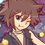# Englishmen sitting in the cinema

I just had a Thai national test yesterday, and I have a question about proving this.

Given $n$ seats,$n \in I^{+} \cup$ {$0$}. And infinite Englishmen.

Prove that the number of ways of Englishmen sitting on the seats is $F_{n+2}$, such that no two Englishmen sit in an adjacent seats (no one sitting is also counted as 1 way).

Example. (0 is vacant. 1 is occupied)

n=0; $\varnothing \rightarrow$ 1 way (no seats, no Englishmen)

n=1; 0 1$\rightarrow$ 2 ways

n=2; 00 01 10 $\rightarrow$ 3 ways

n=3; 000 100 010 001 101 $\rightarrow$ 5 ways

n=4; 0000 1000 0100 0010 0001 1010 1001 0101 $\rightarrow$ 8 ways

n=5; 00000 10000 01000 00100 00010 00001 10100 10010 10001 01010 01001 00101 10101 $\rightarrow$ 13 ways

n=6; 000000 100000 010000 001000 000100 000010 000001 101000 100100 100010 100001 010100 010010 010001 001010 001001 000101 101010 101001 100101 010101$\rightarrow$ 21 ways

etc.....

Note: $F_{n}$ is the nth Fibonacci number, $F_{1} = 1, F_{2} = 1, F_{n} = F_{n-1} + F_{n-2}$ for $n \geq 3$.Note by Samuraiwarm Tsunayoshi
7 years, 4 months ago

This discussion board is a place to discuss our Daily Challenges and the math and science related to those challenges. Explanations are more than just a solution — they should explain the steps and thinking strategies that you used to obtain the solution. Comments should further the discussion of math and science.

When posting on Brilliant:

• Use the emojis to react to an explanation, whether you're congratulating a job well done , or just really confused .
• Ask specific questions about the challenge or the steps in somebody's explanation. Well-posed questions can add a lot to the discussion, but posting "I don't understand!" doesn't help anyone.
• Try to contribute something new to the discussion, whether it is an extension, generalization or other idea related to the challenge.
• Stay on topic — we're all here to learn more about math and science, not to hear about your favorite get-rich-quick scheme or current world events.

MarkdownAppears as
*italics* or _italics_ italics
**bold** or __bold__ bold
- bulleted- list
• bulleted
• list
1. numbered2. list
1. numbered
2. list
Note: you must add a full line of space before and after lists for them to show up correctly
paragraph 1paragraph 2

paragraph 1

paragraph 2

[example link](https://brilliant.org)example link
> This is a quote
This is a quote
    # I indented these lines
# 4 spaces, and now they show
# up as a code block.

print "hello world"
# I indented these lines
# 4 spaces, and now they show
# up as a code block.

print "hello world"
MathAppears as
Remember to wrap math in $$ ... $$ or $ ... $ to ensure proper formatting.
2 \times 3 $2 \times 3$
2^{34} $2^{34}$
a_{i-1} $a_{i-1}$
\frac{2}{3} $\frac{2}{3}$
\sqrt{2} $\sqrt{2}$
\sum_{i=1}^3 $\sum_{i=1}^3$
\sin \theta $\sin \theta$
\boxed{123} $\boxed{123}$

## Comments

Sort by:

Top Newest

I tried proving by strong induction. I can do the induction step, but I can't start the basis step. T__T

I use double counting for the induction step.

Find the number of ways given $n$ seats

1st: nth position is vacant, (n-1) seats remaining, so there're $F_{n+1}$ ways of sitting.

2nd: nth position is occupied, so (n-1)th seat can't have anyone sitting, (n-2) seats remaining, so there're $F_{n}$ ways of sitting.

Therefore, there're $F_{n} + F_{n+1} = F_{n+2}$ ways.

- 7 years, 4 months ago

Log in to reply

Let number of ways of seating $x$ people be $T_x$

To Prove:- $T_n = F_{n+2}$

Then,

Base Case: $T_0 = F_2 = 1 , T_1 = F_3 = 2$

Inductive case : If true for $n, n+1$,

Then, $T_{n} + T_{n+1} = T_{n+2}$ ( Proved in Original Post)

Or, $T_{n} + T_{n+1} = F_{n+2} + F_{n+3} = F_{n+4}$

$\Rightarrow T_{n+2} = F_{n+4}$

- 7 years, 4 months ago

Log in to reply

Thank you ^_^

- 7 years, 4 months ago

Log in to reply

×

Problem Loading...

Note Loading...

Set Loading...